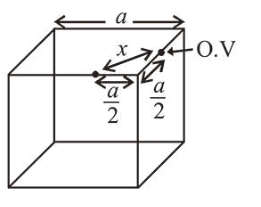# An element crystallises in a face-centred cubic (fcc) unit cell with cell edge a.

Question:

An element crystallises in a face-centred cubic $(f c c)$ unit cell with cell edge $a$. The distance between the centres of two nearest octahedral voids in the crystal lattice is :

1. $\frac{a}{\sqrt{2}}$

2. a

3. $\sqrt{2} a$

4. $\frac{a}{2}$

Correct Option: 1

Solution:Distance between two octahedral voids

$x=\sqrt{\left(\frac{a}{2}\right)^{2}+\left(\frac{a}{2}\right)^{2}}=\sqrt{\frac{a^{2}}{4}+\frac{a^{2}}{4}}=\frac{a}{\sqrt{2}}$